This lesson is in the early stages of development (Alpha version)

# Introduction to Image Manipulation using Nilearn

## Overview

Teaching: 30 min
Exercises: 15 min
Questions
• How can be perform arithmetic operations on MR images

Objectives
• Use Nilearn to perform masking and mathematical operations

• Learn how to resample across modalities for image viewing and manipulation

# Introduction

Nilearn is a functional neuroimaging analysis and visualization library that wraps up a whole bunch of high-level operations (machine learning, statistical analysis, data cleaning, etc…) in easy-to-use commands. The neat thing about Nilearn is that it implements Nibabel under the hood. What this means is that everything you do in Nilearn can be represented by performing a set of operations on Nibabel objects. This has the important consequence of allowing you, yourself to perform high-level operations (like resampling) using Nilearn then dropping into Nibabel for more custom data processing then jumping back up to Nilearn for interactive image viewing. Pretty cool!

# Setting up

The first thing we’ll do is to important some Python modules that will allow us to use Nilearn:

``````import os
import matplotlib.pyplot as plt
from nilearn import image as img
from nilearn import plotting as plot
%matplotlib inline #for inline visualization in jupyter notebook
``````

Notice that we imported two things:

1. `image as img` - allows us to load NIFTI images using nibabel under the hood
2. `plotting as plot`- allows us to using Nilearn’s plotting library for easy visualization

First let’s grab some data from where we downloaded our FMRIPREP outputs:

``````fmriprep_dir='../data/ds000030/derivatives/fmriprep/{subject}/{mod}/'
t1_dir = fmriprep_dir.format(subject='sub-10788', mod='anat')
func_dir = fmriprep_dir.format(subject='sub-10788', mod='func')
``````

We can view the files as follows:

``````os.listdir(t1_dir
``````
``````['sub-10788_T1w_midthickness.L.surf.gii',
'sub-10788_T1w_midthickness.R.surf.gii',
'sub-10788_T1w_preproc.nii.gz',
'sub-10788_T1w_pial.R.surf.gii',
'sub-10788_T1w_space-MNI152NLin2009cAsym_class-GM_probtissue.nii.gz',
'sub-10788_T1w_space-MNI152NLin2009cAsym_class-WM_probtissue.nii.gz',
'sub-10788_T1w_space-MNI152NLin2009cAsym_class-CSF_probtissue.nii.gz',
'sub-10788_T1w_dtissue.nii.gz',
'sub-10788_T1w_inflated.L.surf.gii',
'sub-10788_T1w_smoothwm.R.surf.gii',
'sub-10788_T1w_pial.L.surf.gii',
'sub-10788_T1w_space-MNI152NLin2009cAsym_preproc.nii.gz',
'sub-10788_T1w_smoothwm.L.surf.gii',
'sub-10788_T1w_inflated.R.surf.gii',
'sub-10788_T1w_space-MNI152NLin2009cAsym_warp.h5']
``````

## Warming up with Nilearn

### Basic Image Operations

In this section we’re going to deal with the following files:

1. `sub-10788_T1w_preproc.nii.gz` - the T1 image in native space
2. `sub-10788_T1w_brainmask.nii.gz` - a mask with 1’s representing the brain, and 0’s elsewhere
``````T1 = os.path.join(t1_dir,'sub-10788_T1w_preproc.nii.gz')
``````

We can view our data using Nilearn’s `plotting` module as follows:

``````plot.plot_anat(T1)
``````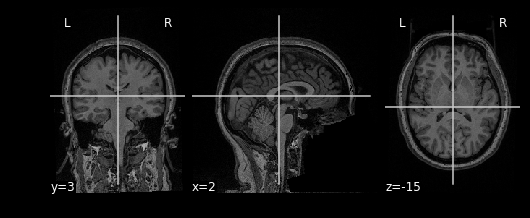Try viewing the mask as well!

Let’s start performing some image operations. The simplest operations we can perform is element-wise, what this means is that we want to perform some sort of mathematical operation on each voxel of the MR image. Since voxels are represented in a 3D array, this is equivalent to performing an operation on each element (i,j,k) of a 3D array. Let’s try inverting the image, that is, flip the colour scale such that all blacks appear white and vice-versa. To do this, we’ll use the method

`img.math_img(formula, **imgs)` Where:

• `formula` is a mathematical expression such as `'a+1'`
• `**imgs` is a set of key-value pairs linking variable names to images. For example `a=T1`

In order to invert the image, we can simply flip the sign which will set the most positive elements (white) to the most negatve elements (black), and the least positives elements (black) to the least negative elements (white). This effectively flips the colour-scale:

``````invert_img = img.math_img('-a', a=T1)
plot.plot_anat(invert_img)
``````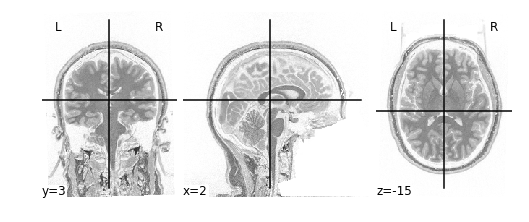Let’s extend this idea of applying operations to each element of an image to multiple images. Instead of specifying just one image like the following:

`img.math_img('a+1',a=img_a)`

We can specify multiple images by tacking on additional variables:

`img.math_img('a+b', a=img_a, b=img_b)`

The key requirement here is that when dealing with multiple images, that the size of the images must be the same. The reason being is that we’re deaing with element-wise operations. That means that some voxel (i,j,k) in `img_a` is being paired with some voxel (i,j,k) in `img_b` when performing operations. So every voxel in `img_a` must have some pair with a voxel in `img_b`; sizes must be the same.

We can take advantage of this property when masking our data using multiplication. Masking works by multipling a raw image (our `T1`), with some mask image (our `bm`). Whichever voxel (i,j,k) has a value of 0 in the mask multiplies with voxel (i,j,k) in the raw image resulting in a product of 0. Conversely, any voxel (i,j,k) in the mask with a value of 1 multiplies with voxel (i,j,k) in the raw image resulting in the same value. Let’s try this out in practice and see what the result is:

``````masked_t1 = img.math_img('a*b', a=T1, b=bm)
``````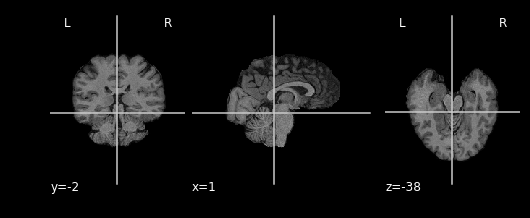As you can see areas where the mask image had a value of 1 were retained, everything else was set to 0

## Exercise #1

Try applying the mask such that the brain is removed, but the rest of the head is intact!

## Solution

``````inverted_mask_t1 = img.math_img('a*(1-b)', a=T1, b=bm)
``````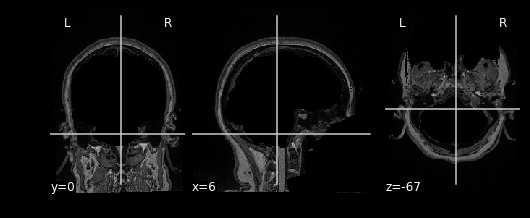### Resampling

Recall from the previous lesson using Nibabel to explore neuroimaging data:

• T1 images are typically composed of voxels that are `1x1x1` in dimension
• Functional images are typically composed of voxels that are larger (`2x2x2` up to `4x4x4`)

This poses problem…

Image that you have two images, one is a `256x256` JPEG image, the other is a `1024x1024` JPEG image. If you were to load up both images into paint, photoshop, or whatever then you can imagine that the first JPEG image would show up to be a lot smaller than the second one. To make it so that both images perfectly overlay each other one thing you could do is to resize the image, maybe by shrinking the larger high-resolution JPEG (1024x1024) down to the smaller low-resolution JPEG.

This JPEG problem is analogous our situation! The T1 image has smaller voxels (higher resolution), and the functional image has larger voxels (lower resolution). Both images represent the same real object and so must be the same size (in mm). Therefore you need more T1 voxels to represent a brain compared to the functional image! You have a mismatch in the dimensions! To fix this issue we need to resize (or more accurately resample) our images so that the dimensions match (same number of voxels).

## Resampling

Resampling is a method of interpolating in between data-points. When we stretch an image we need to figure out what goes in the spaces that are created via stretching - this is what resampling does! Similarily, when we squish an image, we have to toss out some pixels - resampling in this context figures out how to replace values in an image to best represent what the original larger image would have looked like

Let’s implement resampling so that our functional image (called EPI) matches our T1 image.

For this section, we’ll use two new files:

``````mni_T1 = os.path.join(t1_dir,'sub-10788_T1w_space-MNI152NLin2009cAsym_preproc.nii.gz')
``````

Where:

• `mni_T1` now is the standardized T1 image
• `mni_epi` now is the standardized EPI image

First let’s load in our data so we can examine it in more detail, remember Nilearn will load in the image as a nibabel object:

``````mni_t1_img = img.load_img(mni_T1)
print("Data is type", type(mni_t1_img))
print("T1 dimensions", mni_t1_img.shape)
print("EPI dimensions", mni_epi_img.shape)
``````
``````Data is type	<class 'nibabel.nifti1.Nifti1Image'>
T1 dimensions	(193, 229, 193)
EPI dimensions	(65, 77, 49, 152)
``````

This confirms our theory that the T1 image has a lot more voxels than the EPI image. Note that the 4th dimension of the EPI image are timepoints which we can safely ignore for now.

We can resample an image using nilearn’s `img.resample_to_img` function, which has the following structure:

`img.resample_to_img(source_img,target_img,interpolation)`

• `source_img` the image you want to sample
• `target_img` the image you wish to resample to
• `interpolation` the method of interpolation

## Interpolation

Nilearn supports 3 types of interpolation, the one you’ll use depends on the type of data you’re resampling!

1. continuous - Interpolate but maintain some edge features. Ideal for structural images where edges are well-defined. Uses 3rd-order spline interpolation.
2. linear (default) - Interpolate uses a combination of neighbouring voxels - will blur. Uses trilinear interpolation.
3. nearest - matches value of closest voxel (majority vote from neighbours). This is ideal for masks which are binary since it will preserve the 0’s and 1’s and will not produce in-between values (ex: 0.342). Also ideal for numeric labels where values are 0,1,2,3… (parcellations). Uses nearest-neighbours interpolation with majority vote.

Let’s implement this:

``````resamp_mni_T1 = img.resample_to_img(source_img=mni_t1_img, target_img=mni_epi_img, interpolation='continuous')
print("Resampled T1 dimensions", resamp_mni_T1.shape)
print("EPI dimensions", mni_epi_img.shape)
``````
``````Resampled T1 dimensions		(65, 77, 49)
EPI dimensions			(65, 77, 49, 152)
``````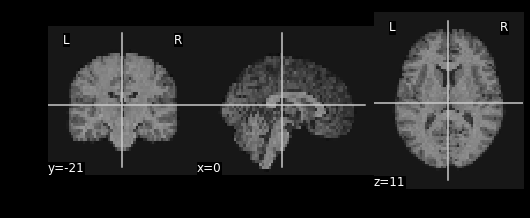As you might notice, we have a blockier version of our T1 image – we’ve reduce the resolution to match that of the EPI image.

## Challenge

Using the Native T1 and Resting State in T1 space do the following:

1. Resample the Native T1 to match the Resting State image
2. Replace the brain in the T1 image with the first frame of the resting state brain

Some files you’ll need

``````ex_T1 = os.path.join(t1_dir,'sub-10788_T1w_preproc.nii.gz')
``````

## Solution

``````#Resample
resamp_t1 = img.resample_to_img(source_img=ex_T1,target_img=ex_func,interpolation='continuous')

#Step 2: We need to resample the mask as well!
resamp_bm = img.resample_to_img(source_img=ex_bm,target_img=resamp_t1,interpolation='nearest')

#Step 3: Mask out the T1 image
removed_t1 = img.math_img('a*(1-b)',a=resamp_t1,b=resamp_bm)

#Visualize the resampled and removed brain
plot.plot_anat(removed_t1)

``````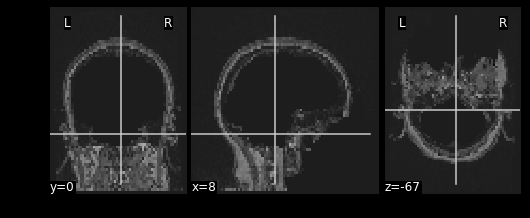``````#Load in the first frame of the resting state image
first_func_img = func_img.slicer[:,:,:,0]

#Mask the functional image and visualize
``````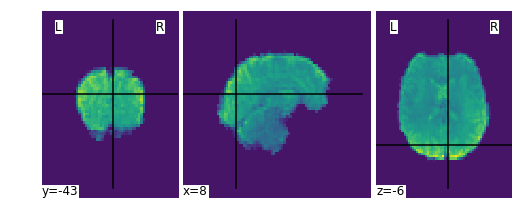#Now overlay the functional image on top of the anatomical missing the brain

``````combined_img = img.math_img('a+b', a=removed_t1, b=masked_func)
plot.plot_anat(combined_img)
``````## Key Points

• MR images are essentially 3D arrays where each voxel is represented by an (i,j,k) index

• Nilearn is Nibabel under the hood, knowing how Nibabel works is key to understanding Nilearn